# 7.3: What does F mean?

$$\newcommand{\vecs}{\overset { \rightharpoonup} {\mathbf{#1}} }$$ $$\newcommand{\vecd}{\overset{-\!-\!\rightharpoonup}{\vphantom{a}\smash {#1}}}$$$$\newcommand{\id}{\mathrm{id}}$$ $$\newcommand{\Span}{\mathrm{span}}$$ $$\newcommand{\kernel}{\mathrm{null}\,}$$ $$\newcommand{\range}{\mathrm{range}\,}$$ $$\newcommand{\RealPart}{\mathrm{Re}}$$ $$\newcommand{\ImaginaryPart}{\mathrm{Im}}$$ $$\newcommand{\Argument}{\mathrm{Arg}}$$ $$\newcommand{\norm}{\| #1 \|}$$ $$\newcommand{\inner}{\langle #1, #2 \rangle}$$ $$\newcommand{\Span}{\mathrm{span}}$$ $$\newcommand{\id}{\mathrm{id}}$$ $$\newcommand{\Span}{\mathrm{span}}$$ $$\newcommand{\kernel}{\mathrm{null}\,}$$ $$\newcommand{\range}{\mathrm{range}\,}$$ $$\newcommand{\RealPart}{\mathrm{Re}}$$ $$\newcommand{\ImaginaryPart}{\mathrm{Im}}$$ $$\newcommand{\Argument}{\mathrm{Arg}}$$ $$\newcommand{\norm}{\| #1 \|}$$ $$\newcommand{\inner}{\langle #1, #2 \rangle}$$ $$\newcommand{\Span}{\mathrm{span}}$$$$\newcommand{\AA}{\unicode[.8,0]{x212B}}$$

We’ve just noted that the ANOVA has a bunch of numbers that we calculated straight from the data. All except one, the $$p$$-value. We did not calculate the $$p$$-value from the data. Where did it come from, what does it mean? How do we use this for statistical inference. Just so you don’t get too worried, the $$p$$-value for the ANOVA has the very same general meaning as the $$p$$-value for the $$t$$-test, or the $$p$$-value for any sample statistic. It tells us that the probability that we would observe our test statistic or larger, under the distribution of no differences (the null).

As we keep saying, $$F$$ is a sample statistic. Can you guess what we do with sample statistics in this textbook? We did it for the Crump Test, the Randomization Test, and the $$t$$-test… We make fake data, we simulate it, we compute the sample statistic we are interested in, then we see how it behaves over many replications or simulations.

Let’s do that for $$F$$. This will help you understand what $$F$$ really is, and how it behaves. We are going to created the sampling distribution of $$F$$. Once we have that you will be able to see where the $$p$$-values come from. It’s the same basic process that we followed for the $$t$$ tests, except we are measuring $$F$$ instead of $$t$$.

Here is the set-up, we are going to run an experiment with three levels. In our imaginary experiment we are going to test whether a new magic pill can make you smarter. The independent variable is the number of magic pills you take: 1, 2, or 3. We will measure your smartness using a smartness test. We will assume the smartness test has some known properties, the mean score on the test is 100, with a standard deviation of 10 (and the distribution is normal).

The only catch is that our magic pill does NOTHING AT ALL. The fake people in our fake experiment will all take sugar pills that do absolutely nothing to their smartness. Why would we want to simulate such a bunch of nonsense? The answer is that this kind of simulation is critical for making inferences about chance if you were to conduct a real experiment.

Here are some more details for the experiment. Each group will have 10 different subjects, so there will be a total of 30 subjects. We are going to run this experiment 10,000 times. Each time drawing numbers randomly from the very same normal distribution. We are going to calculate $$F$$ from our sample data every time, and then we are going to draw the histogram of $$F$$-values. This will show us the sampling distribution of $$F$$ for our situation. Let’s do that and see what it looks like: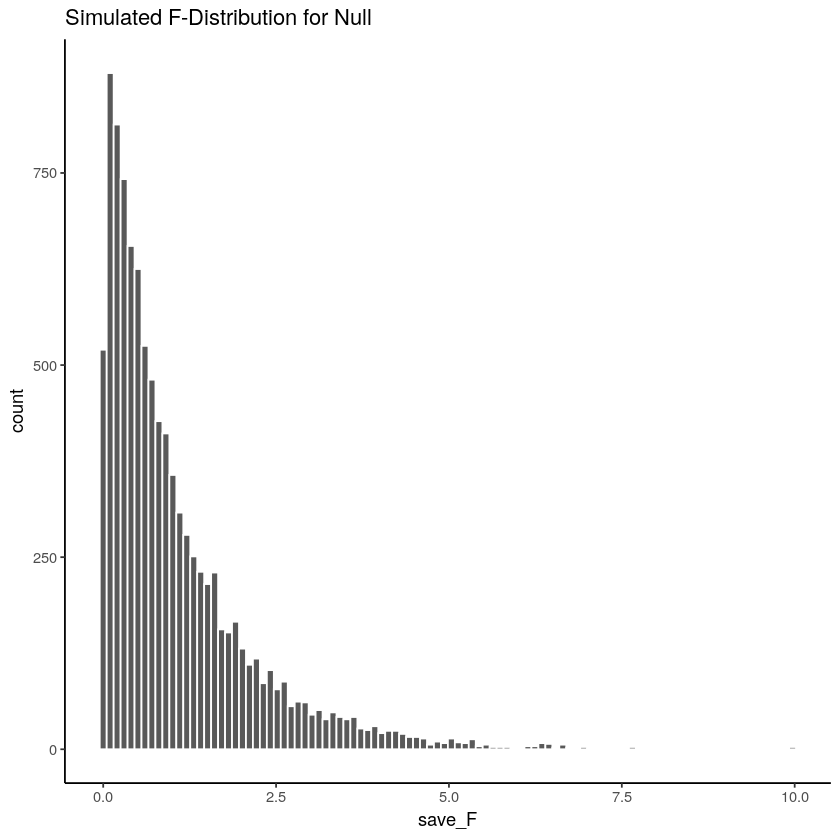Figure $$\PageIndex{1}$$: A simulation of 10,000 experiments from a null distribution where there is no differences. The histogram shows 10,000 $$F$$-values, one for each simulation. These are values that F can take in this situation. All of these $$F$$-values were produced by random sampling error.

Let’s note a couple things about the $$F$$ distribution. 1) The smallest value is 0, and there are no negative values. Does this make sense? $$F$$ can never be negative because it is the ratio of two variances, and variances are always positive because of the squaring operation. So, yes, it makes sense that the sampling distribution of $$F$$ is always 0 or greater. 2) it does not look normal. No it does not. $$F$$ can have many different looking shapes, depending on the degrees of freedom in the numerator and denominator. However, these aspects are too important for now.

Remember, before we talked about some intuitive ideas for understanding $$F$$, based on the idea that $$F$$ is a ratio of what we can explain (variance due to mean differences), divided by what we can’t explain (the error variance). When the error variance is higher than the effect variance, then we will always get an $$F$$-value less than one. You can see that we often got $$F$$-values less than one in the simulation. This is sensible, after all we were simulating samples coming from the very same distribution. On average there should be no differences between the means. So, on average the part of the total variance that is explained by the means should be less than one, or around one, because it should be roughly the same as the amount of error variance (remember, we are simulating no differences).

At the same time, we do see that some $$F$$-values are larger than 1. There are little bars that we can see going all the way up to about 5. If you were to get an $$F$$-value of 5, you might automatically think, that’s a pretty big $$F$$-value. Indeed it kind of is, it means that you can explain 5 times more of variance than you can’t explain. That seems like a lot. You can also see that larger $$F$$-values don’t occur very often. As a final reminder, what you are looking at is how the $$F$$-statistic (measured from each of 10,000 simulated experiments) behaves when the only thing that can cause differences in the means is random sampling error. Just by chance sometimes the means will be different. You are looking at another chance window. These are the $$F$$s that chance can produce.

## Making Decisions

We can use the sampling distribution of $$F$$ (for the null) to make decisions about the role of chance in a real experiment. For example, we could do the following.

1. Set an alpha criterion of $$p$$ = 0.05
2. Find out the critical value for $$F$$, for our particular situation (with our $$df$$s for the numerator and denominator).

Let’s do that. I’ve drawn the line for the critical value onto the histogram: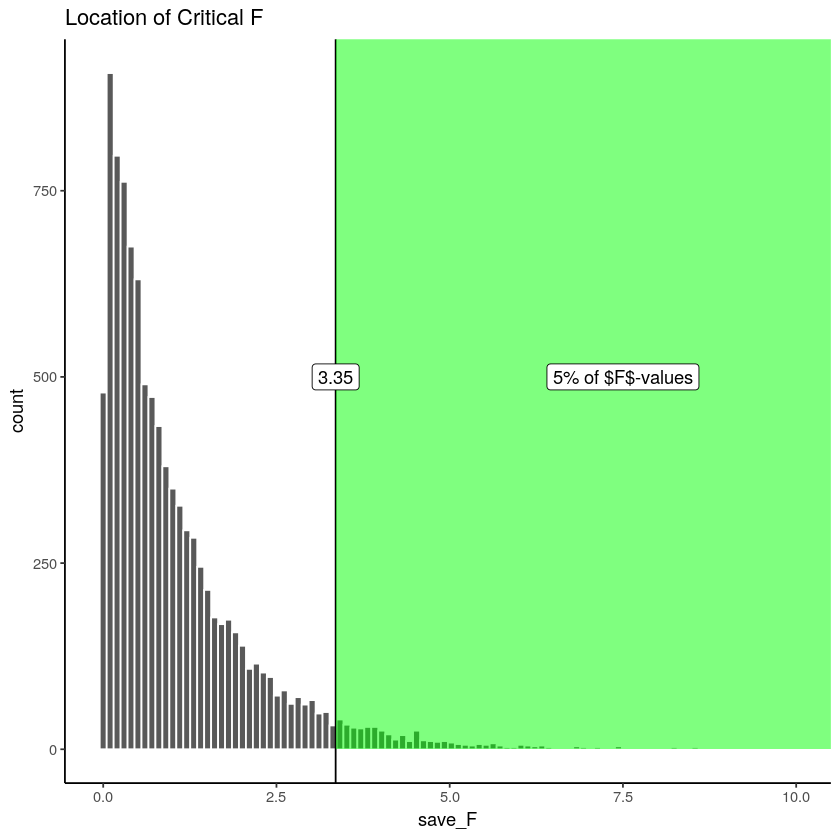Figure $$\PageIndex{2}$$: The critical value for F where 5% of all $$F$$-values lie beyond this point.

Alright, now we can see that only 5% of all $$F$$-values from from this sampling distribution will be 3.35 or larger. We can use this information.

How would we use it? Imagine we ran a real version of this experiment. And, we really used some pills that just might change smartness. If we ran the exact same design, with 30 people in total (10 in each group), we could set an $$F$$ criterion of 3.35 for determining whether any of our results reflected a causal change in smartness due to the pills, and not due to random chance. For example, if we found an $$F$$-value of 3.34, which happens, just less than 5% of the time, we might conclude that random sampling error did not produce the differences between our means. Instead, we might be more confident that the pills actually did something, after all an $$F$$-value of 3.34 doesn’t happen very often, it is unlikely (only 5 times out of 100) to occur by chance.

## Fs and means

Up to here we have been building your intuition for understanding $$F$$. We went through the calculation of $$F$$ from sample data. We went through the process of simulating thousands of $$F$$s to show you the null distribution. We have not talked so much about what researchers really care about…The MEANS! The actual results from the experiment. Were the means different? that’s often what people want to know. So, now we will talk about the means, and $$F$$, together.

Notice, if I told you I ran an experiment with three groups, testing whether some manipulation changes the behavior of the groups, and I told you that I found a big $$F$$!, say an $$F$$ of 6!. And, that the $$F$$ of 6 had a $$p$$-value of .001. What would you know based on that information alone? You would only know that Fs of 6 don’t happen very often by chance. In fact they only happen 0.1% of the time, that’s hardly at all. If someone told me those values, I would believe that the results they found in their experiment were not likely due to chance. However, I still would not know what the results of the experiment were! Nobody told us what the means were in the different groups, we don’t know what happened!

IMPORTANT: even though we don’t know what the means were, we do know something about them, whenever we get $$F$$-values and $$p$$-values like that (big $$F$$s, and very small associated $$p$$s)… Can you guess what we know? I’ll tell you. We automatically know that there must have been some differences between the means. If there was no differences between the means, then the variance explained by the means (the numerator for $$F$$) would not be very large. So, we know that there must be some differences, we just don’t know what they are. Of course, if we had the data, all we would need to do is look at the means for the groups (the ANOVA table doesn’t report this, we need to do it as a separate step).

### ANOVA is an omnibus test

This property of the ANOVA is why the ANOVA is sometimes called the omnibus test. Omnibus is a fun word, it sounds like a bus I’d like to ride. The meaning of omnibus, according to the dictionary, is “comprising several items”. The ANOVA is, in a way, one omnibus test, comprising several little tests.

For example, if you had three groups, A, B, and C. You get could differences between

1. A and B
2. B and C
3. A and C

That’s three possible differences you could get. You could run separate $$t$$-tests, to test whether each of those differences you might have found could have been produced by chance. Or, you could run an ANOVA, like what we have been doing, to ask one more general question about the differences. Here is one way to think about what the omnibus test is testing:

Hypothesis of no differences anywhere: $$A = B = C$$

Any differences anywhere:

1. $$A \neq B = C$$
2. $$A = B \neq C$$
3. $$A \neq C = B$$

The $$\neq$$ symbol means “does not equal”, it’s an equal sign with a cross through it (no equals allowed!).

How do we put all of this together. Generally, when we get a small $$F$$-value, with a large $$p$$-value, we will not reject the hypothesis of no differences. We will say that we do not have evidence that the means of the three groups are in any way different, and the differences that are there could easily have been produced by chance. When we get a large F with a small $$p$$-value (one that is below our alpha criterion), we will generally reject the hypothesis of no differences. We would then assume that at least one group mean is not equal to one of the others. That is the omnibus test. Rejecting the null in this way is rejecting the idea there are no differences. But, the $$F$$ test still does not tell you which of the possible group differences are the ones that are different.

### Looking at a bunch of group means

We ran 10,000 experiments just before, and we didn’t even once look at the group means for any of the experiments. Let’s quickly do that, so we get a better sense of what is going on.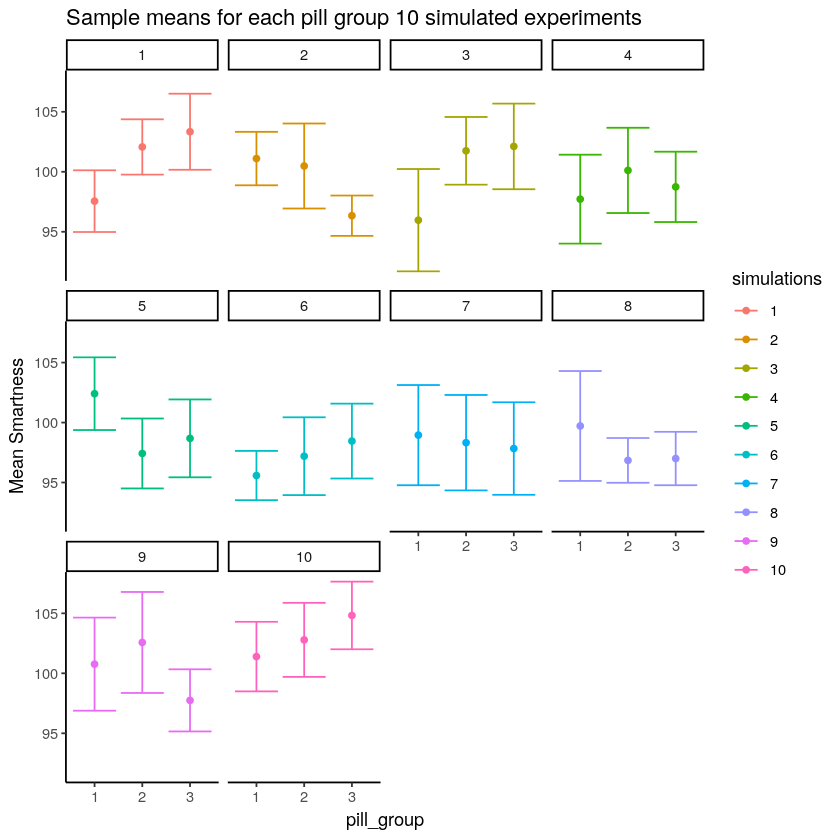Figure $$\PageIndex{3}$$: Different patterns of group means under the null (all scores for each group sampled from the same distribution).

Whoa, that’s a lot to look at. What is going on here? Each little box represents the outcome of a simulated experiment. The dots are the means for each group (whether subjects took 1 , 2, or 3 magic pills). The y-axis shows the mean smartness for each group. The error bars are standard errors of the mean.

You can see that each of the 10 experiments turn out different. Remember, we sampled 10 numbers for each group from the same normal distribution with mean = 100, and sd = 10. So, we know that the correct means for each sample should actually be 100 every single time. However, they are not 100 every single time because of?…sampling error (Our good friend that we talk about all the time).

For most of the simulations the error bars are all overlapping, this suggests visually that the means are not different. However, some of them look like they are not overlapping so much, and this would suggest that they are different. This is the siren song of chance (sirens lured sailors to their deaths at sea…beware of the siren call of chance). If we concluded that any of these sets of means had a true difference, we would be committing a type I error. Because we made the simulation, we know that none of these means are actually different. But, when you are running a real experiment, you don’t get to know this for sure.

### Looking at bar graphs

Let’s look at the exact same graph as above, but this time use bars to visually illustrate the means, instead of dots. We’ll re-do our simulation of 10 experiments, so the pattern will be a little bit different: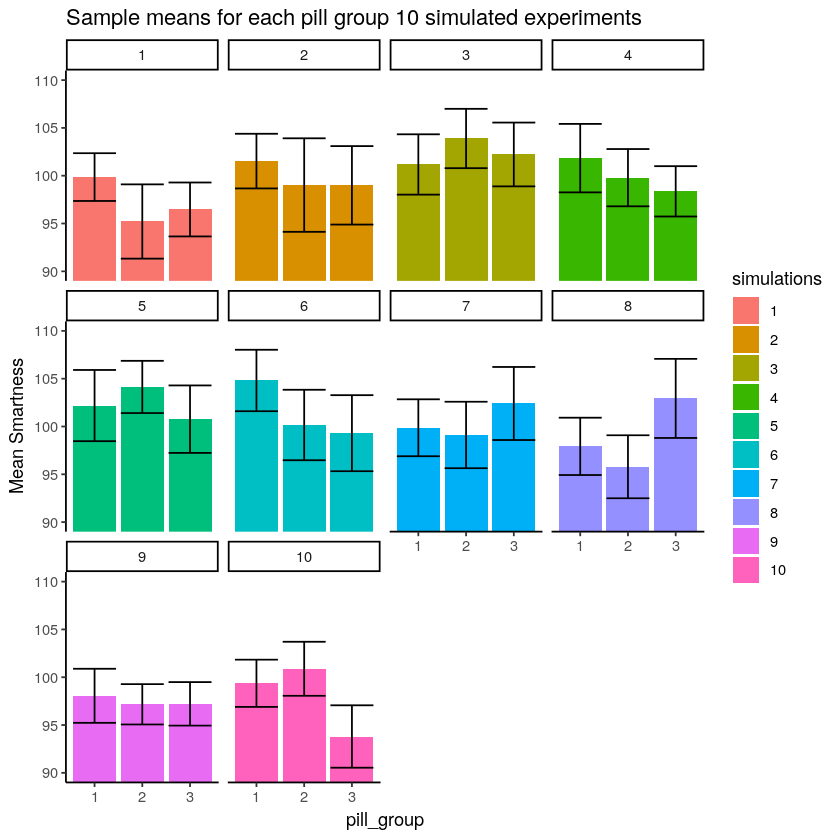Figure $$\PageIndex{4}$$: Different patterns of group means under the null (all scores for each group sampled from the same distribution).

Now the heights of the bars display the means for each pill group. In general we see the same thing. Some of the fake experiments look like there might be differences, and some of them don’t.

### What mean differences look like when F is < 1

We are now giving you some visual experience looking at what means look like from a particular experiment. This is for your stats intuition. We’re trying to improve your data senses.

What we are going to do now is similar to what we did before. Except this time we are going to look at 10 simulated experiments, where all of the $$F$$-values were less than 1. All of these $$F$$-values would also be associated with fairly large $$p$$-values. When F is less than one, we would not reject the hypothesis of no differences. So, when we look at patterns of means when F is less than 1, we should see mostly the same means, and no big differences.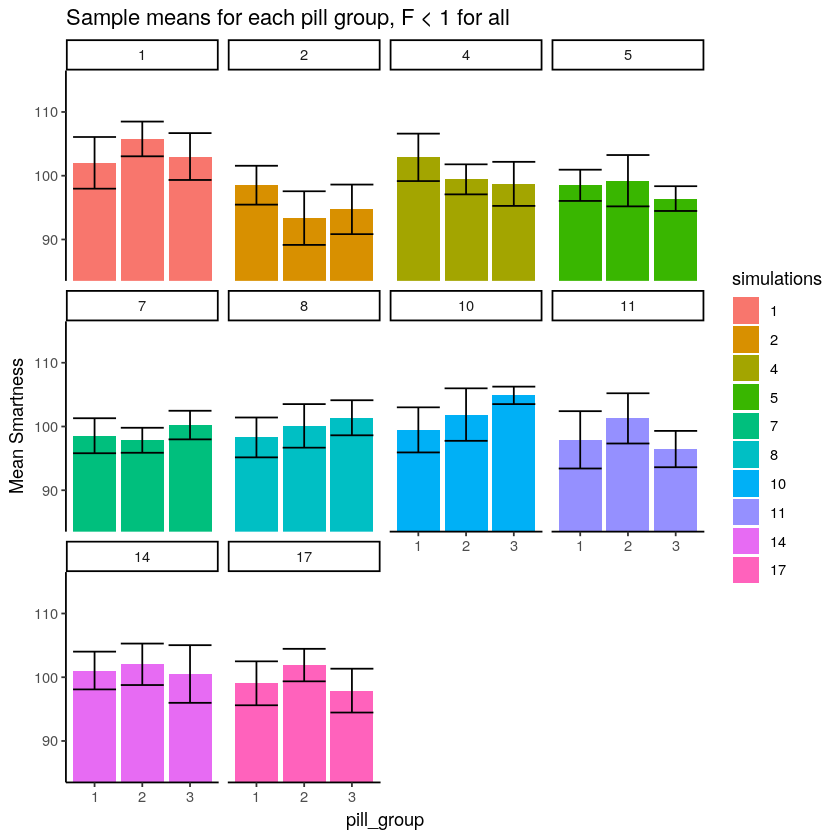Figure $$\PageIndex{5}$$: Different patterns of group means under the null (sampled from same distribution) when F is less than 1.

The numbers in the panels now tell us which simulations actually produced Fs of less than 1.

We see here that all the bars aren’t perfectly flat, that’s OK. What’s more important is that for each panel, the error bars for each mean are totally overlapping with all the other error bars. We can see visually that our estimate of the mean for each sample is about the same for all of the bars. That’s good, we wouldn’t make any type I errors here.

### What mean differences look like when F > 3.35

Earlier we found that the critical value for $$F$$ in our situation was 3.35, this was the location on the $$F$$ distribution where only 5% of $$F$$s were 3.35 or greater. We would reject the hypothesis of no differences whenever $$F$$ was greater than 3.35. In this case, whenever we did that, we would be making a type I error. That is because we are simulating the distribution of no differences (remember all of our sample means are coming from the exact same distribution). So, now we can take a look at what type I errors look like. In other words, we can run some simulations and look at the pattern in the means, only when F happens to be 3.35 or greater (this only happens 5% of the time, so we might have to let the computer simulate for a while). Let’s see what that looks like: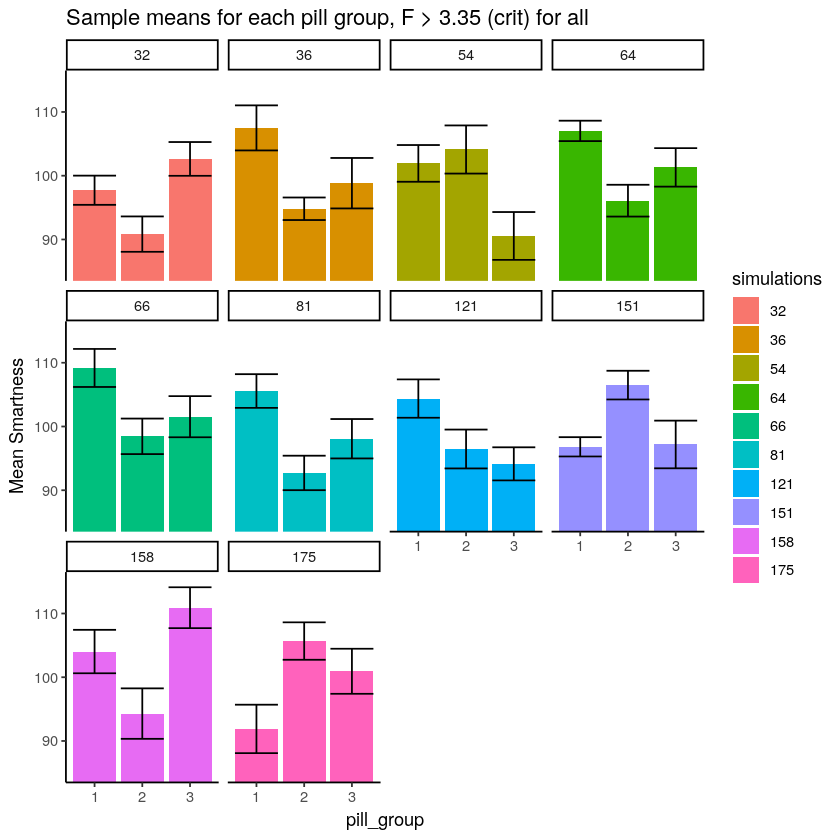Figure $$\PageIndex{6}$$: Different patterns of group means under the null when F is above critical value (these are all type I Errors).

The numbers in the panels now tell us which simulations actually produced $$F$$s that were greater than 3.35

What do you notice about the pattern of means inside each panel? Now, every single panel shows at least one mean that is different from the others. Specifically, the error bars for one mean do not overlap with the error bars for one or another mean. This is what mistakes looks like. These are all type I errors. They are insidious. When they happen to you by chance, the data really does appear to show a strong pattern, and your $$F$$-value is large, and your $$p$$-value is small! It is easy to be convinced by a type I error (it’s the siren song of chance).

This page titled 7.3: What does F mean? is shared under a CC BY-SA 4.0 license and was authored, remixed, and/or curated by Matthew J. C. Crump via source content that was edited to the style and standards of the LibreTexts platform; a detailed edit history is available upon request.